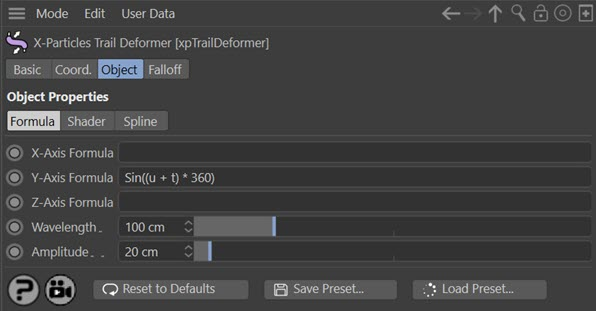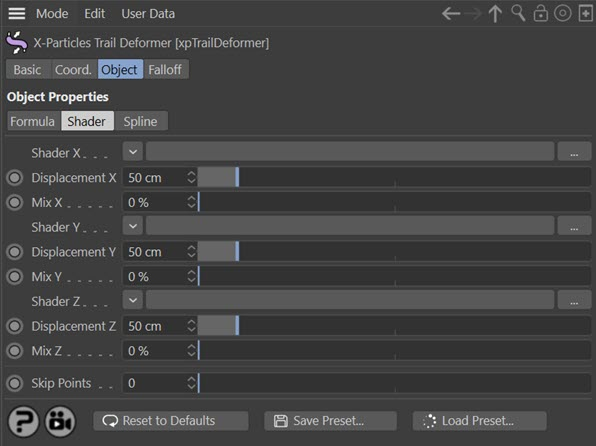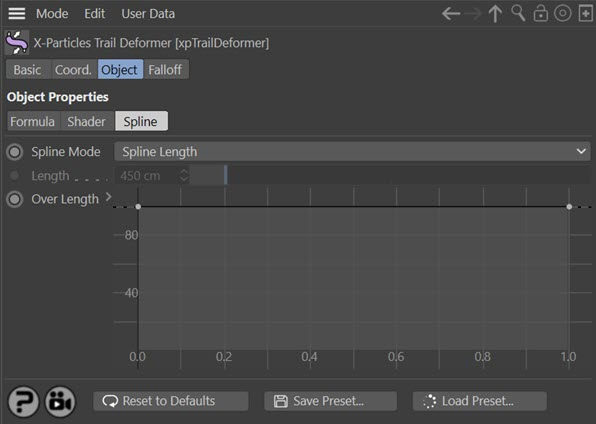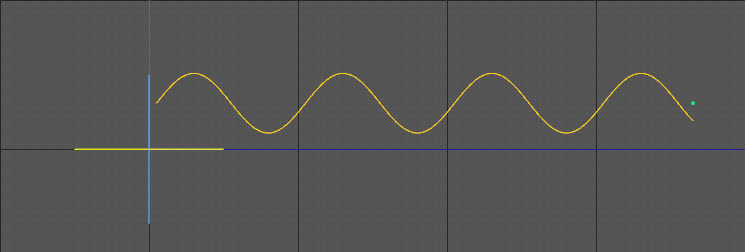# Trail Deformer

This deformer is used in the same way as any other deformer in Cinema 4D, but acts only on spline objects. You can use it on any spline but it is specifically intended for use with the X-Particles Trail object.

Note that this deformer does not affect particle movement at all, it only affects the generated spline. To use it, simply make it a child object of the Trail object (or other spline).

Please see the section 'Using the Trail Deformer' before using this object for the first time.

## Interface

The object's interface includes the following sections:

For the 'Falloff' tab and the buttons at the bottom of the interface, please see the 'Common interface elements' page.

## Formula quicktab### X-Axis Formula, Y-Axis Formula, Z-Axis Formula

The formulas to use for displacement along the X and Y axis of the spline. An empty field will have no effect.

### Wavelength

A value indicating how often a pattern will repeat. It is important to note that this parameter will have NO EFFECT unless the symbol 'u' (see below) is present in a formula. If it is not present, changing this value will not change the pattern repeat.

### Amplitude

The amount of displacement. This is multiplied by the result of the formula to find the actual displacement of a point.The shaders to use for shader-based displacement of a point. An empty link field will have no effect on the point. The shader is sampled for each point on the trail spline, typically this will return a value between 0 and 1 for each point .

### Displacement X, Displacement Y, Displacement Z

The amount of displacement generated by the shader along each axis. This is multiplied by the result of sampling the shaders to find the actual displacement of a point.

### Mix X, Mix Y, Mix Z

The contribution to the displacement made by the formulas and shaders. A value of 0% means that all the displacement is generated by the formulas; a value of 100% means that the shaders control the displacement and the formulas have no effect.

Note that the default value is 0%, so even if you add shaders to the link fields, you will see no effect until you increase the 'Mix' value.

### Skip Points

If this setting is greater than zero, the deformer will skip that many vertices in the spline. In other words, if it is set to 1, every other vertex will not be affected by the deformer.

## Spline quicktab### Spline Mode

A drop-down menu with two options, Spline Length and Fixed Length. These are explained in the Spline Control section above.

### Length

The fixed-length value to use when 'Spline Mode' is set to 'Fixed Length'.

### Over Length

The actual control spline. See the 'Spline Control' section above.

## Using the Trail Deformer

### Formula Displacement

This is the core of the deformer. It functions in a similar way to the Formula Deformer that comes with Cinema, but with some differences.

The way the deformer works is that for each point in the spline a displacement along one or more of the axes is calculated and added to the current spline point coordinate. An example would be the simplest possible formula, consisting of a single number - let's say, '2'. So you would type '2' into the formula fields.

The deformer takes that value and multiplies it by the value in the 'Amplitude' parameter. If that is the default of 20 units, the result is 2 x 20 = 40. The value '40' is then added to the point's coordinate value. If this was added to the Y coordinate, the effect would be to move all the points in the spline along the positive Y axis by 40 units.

That isn't very useful, but with more complex formulas you can do a lot more than that. The default formula in the deformer is along the Y axis of the point and reads:

##### Sin((u + t) * 360)

A very simple formula, but the effect is to displace the spline along to Y to produce a sine wave:You can use all the mathematical functions supported by Cinema 4D (see the Appendix in Cinema's online help for details) and can also use a few special symbols added by this deformer. These are explained below.

#### Formula symbols

As well as the functions and symbols implicitly supported by Cinema 4D, details of which can be found in Cinema's online help, this deformer supports additional symbols that you can use in your formulas. These are as follows.

Symbol Meaning
t The scene time in seconds.
x The current value of the X-coordinate of a spline point, in object space.
y The current value of the Y-coordinate of a spline point, in object space.
z The current value of the Z-coordinate of a spline point, in object space.
w The wavelength value from the deformer's interface.
a The amplitude value from the deformer's interface.
r A value from 0 to 1 representing the relative position of a trail spline point along the length of the spline. The first point will always return a value of 0; the last point a value of 1. For all other points the value will change as the trail spline gets longer.
u A value from 0 to 1 which is the position of a spline point in terms of a proportion of one wavelength. For example, if the wavelength is 100 units and a point is 50 units from the start of the spline, the 'u' value is 50/100 = 0.5. If the point is 175 units from the start, the 'u' value is 175/100 = 1.75, but values of more than 1 are reduced to a value of 0 to 1, so the actual 'u' value is 0.75 (and would also be 0.75 for points 275, 375, 475 etc. units from the start of the spline). This permits repeating patterns to be created, as shown in the sine wave above.

Remember that in all cases the value returned from a formula is then multiplied by the Amplitude value to give the actual displacement.

#### Formulas for displacement on different axes

You can use different formulas for the formula fields, or use the same formula in both (which is often not very useful) or only use a formula for one axis. An empty formula field has no effect on the spline point.

#### Other notes

There are three points to note:

1. If the formula field is empty, it returns a value of zero. Since this is then multiplied by the amplitude, this gives a displacement of zero along that axis (i.e. no effect). The same result would be achieved by entering the value '0' in the formula field.

2. Errors in the formula also return zero. If your formula appears to have no effect, it may have an error such as a symbol that isn't recognised or an imbalance in the number of parentheses used in the formula.

3. If you need to include angles in the formula, these are always in degrees (e.g. enter '360' not 'pi * 2' if you want an angle of 360 degrees).

You can displace the trail spline points using a shader as well as, or instead of, a formula. The amount of displacement is given independently in the 'Displacement' parameter. Any shader can be used, including animated ones. Some, of course, will be much more useful than others. The one which you will probably use most is 'Noise'.

The blend between formula and shader displacement is controlled by the 'Mix' parameter. By default this is set to 0%, which means no contribution from the shader (if any) and the displacement is entirely due to the formula (if there is one). A value of 100% means that the shader controls the displacement completely, with no effect from the formula.

### Spline Control

In addition to the above, you can also control the displacement over the length of the spline with the spline control. This enables you to do things such as having no displacement at the start of the spline and maximum displacement at the end; with a sine wave formula this gives a tentacle-like effect.

The spline works by multiplying the calculated displacement from the formulas and/or shaders by the value from the spline control; this value in turn depends on the relative position of a trail spline point along the length of the trail spline. The first point on the trail spline will always use the value from the extreme left of the control spline.

For all points on the trail spline, the value obtained from the control spline in the interface depends on the relative position of the point along the trail length. But what is meant by the 'trail length'? Since the trail may get longer over time, the length can be regarded in one of two ways. If the 'Spline Mode' parameter is set to 'Fixed Length' then the relative position of each point is calculated according to the value in the 'Length' parameter. For example, if 'Length' is set to 450 units, and a point on the trail spline is located at 150 units from the start of the trail, the point has a relative value of 150/450 = 0.333. This is then used to read the value from control spline. Note that in this mode the relative value will never change for a given point.

If 'Spline Mode' is set to 'Spline Length' then the actual length of the trail spline is used. So, if at a specific time a point on the trail spline is 50 units from the trail start and the length of the trail at that point is 100 units, the relative position is 50/100 = 0.5. But if after some more time has elapsed the trail length is now 200 units, the relative position of the point will have changed - it is now 50/200 = 0.25.

Different effects will result from these two modes.